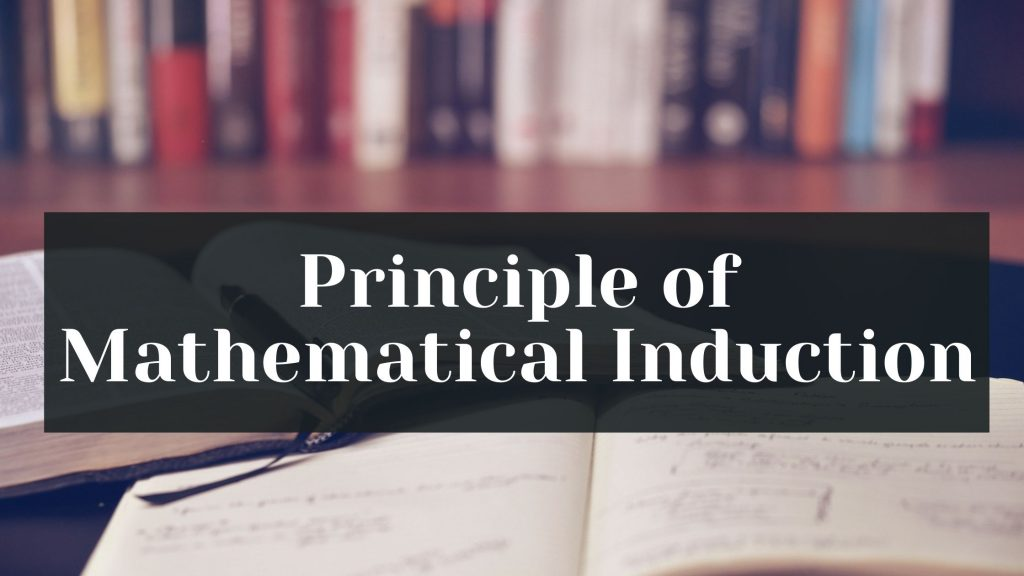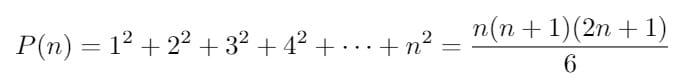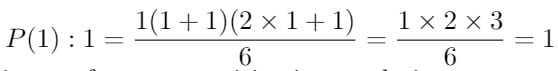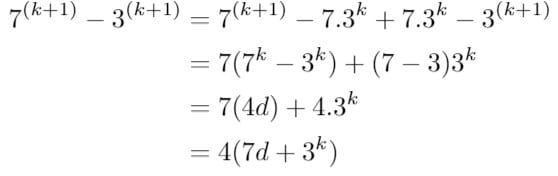Maths
Study Material

# Principle of Mathematical Induction and Solved Examples

Principle of Mathematical Induction is used in several fields of mathematics to prove statements of the form P(n), n ∈ N. Let us learn about it.

4 minutes long
Posted by Tanwir Silar, 18/7/2021Hesap Oluştur

Got stuck on homework? Get your step-by-step solutions from real tutors in minutes! 24/7. Unlimited.

Let us get familiar with the tool of mathematics which helps us to prove or disprove a statement of the form P(n), n ∈ N. that is Principle of Mathematical Induction.

## What is an Inductive Step?

Before going through the formal definition of the concept, it is better to know about an inductive set. A set S is said to be an inductive set if 1∈ S and x + 1 ∈ S whenever x ∈ S. Since N is the smallest subset of R which is an inductive set, it follows that any subset of R that is an inductive set must contain N.

## Generic solution approach

Having known about the inductive set, let us move forward by discussing the approach to solve a problem of Mathematical Induction. Suppose there is a given statement P(n) involving the natural number n such that

(i) The statement is true for n = 1, i.e., P(1) is true, and
(ii) If the statement is true for n = k (where k is some positive integer), then the statement is also true for n = k+1, i.e., truth of P(k) implies the truth of P(k+1).

Then, P(n) is true for all natural numbers n.

The second step of the approach is called the inductive hypothesis, while the first step is often referred as the basic step.

## Solved Problems on Mathematical Induction

Example 1: For all n ≥ 1, prove that

Solution: Let the given statement be P(n) i.e.,Step 1: Prove that P(1) is true
For n = 1,, which is true.

Step 2: Assume that P(k) is true for some positive integer k i.e.,

We shall now prove that P(k + 1) is also true. Now, we have

Thus P(k + 1) is true, whenever P(k) is true.
Hence, from the principle of mathematical induction, the statement P(n) is true for all natural numbers n.

Example 2: For every positive integer n, prove that 7n – 3n is divisible by 4.

Solution: We can write
P(n) : 7n – 3n is divisible by 4

Step 1:
We need to prove P(1) is true
P(1): 7 – 3 = 4 which is divisible by 4. Thus P(n) is true for n = 1
Step 2:
Let P(k) be true for some natural number k,
i.e., P(k) : 7k – 3k is divisible by 4.
We can write, 7k – 3k = 4d where d ∈ N.
As part of the induction hypothesis, we need to prove that P(k + 1) is true whenever P(k) is true.
Now, we can write P(k+1) as,The above calculations show that 7(k+1) – 3(k+1) is divisible by 4. Thus, P(k+1) is true when P(k) is true.
Hence, from the principle of mathematical induction, the statement P(n) is true for all natural numbers n.

Any problem related to Principle of Mathematical Induction could be solved using the above approach.